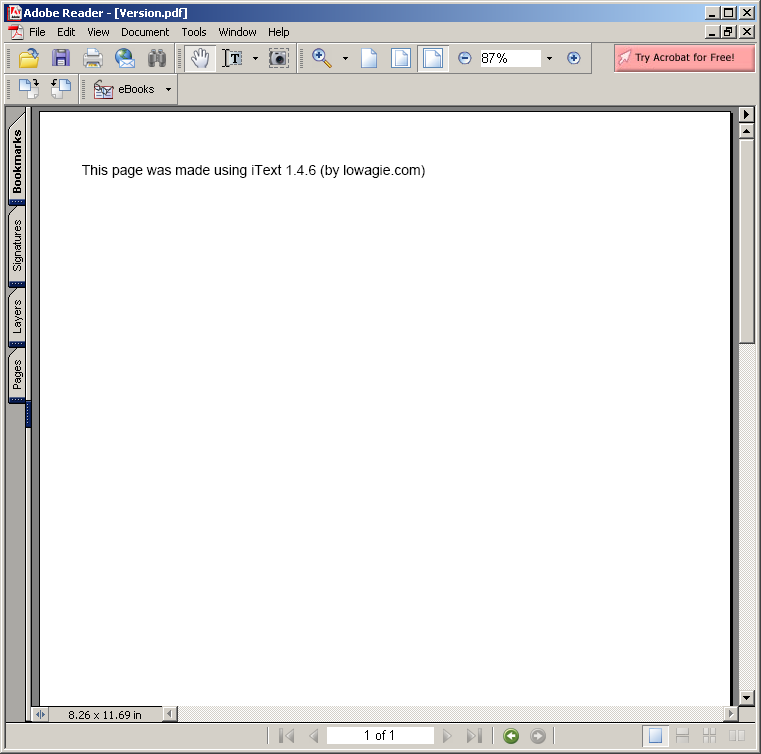# Fibonacci : Algorithms « Collections Data Structure « Java

Fibonacci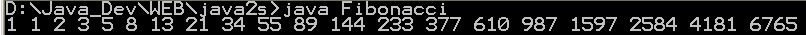```
/*
* This code is from the book Java Examples in a Nutshell, 2nd Edition.
* It is provided AS-IS, WITHOUT ANY WARRANTY either expressed or implied.
* You may study, use, and modify it for any non-commercial purpose.
* You may distribute it non-commercially as long as you retain this notice.
* For a commercial use license, or to purchase the book (recommended),
* visit http://www.davidflanagan.com/javaexamples2.
*/

/**
* This program prints out the first 20 numbers in the Fibonacci sequence. Each
* term is formed by adding together the previous two terms in the sequence,
* starting with the terms 1 and 1.
*/
public class Fibonacci {
public static void main(String[] args) {
int n0 = 1, n1 = 1, n2; // Initialize variables
System.out.print(n0 + " " + // Print first and second terms
n1 + " "); // of the series

for (int i = 0; i < 18; i++) { // Loop for the next 18 terms
n2 = n1 + n0; // Next term is sum of previous two
System.out.print(n2 + " "); // Print it out
n0 = n1; // First previous becomes 2nd previous
n1 = n2; // And current number becomes previous
}
System.out.println(); // Terminate the line
}
}

```

### Related examples in the same category

 1 Anagrams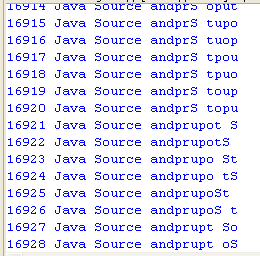2 Hanoi puzzle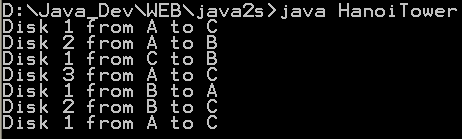3 Sieve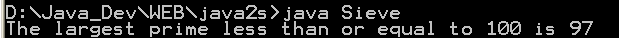4 Find connections using a depth-first search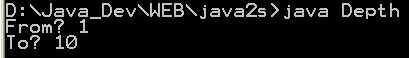5 Find connections using hill climbing. 6 Find optimal solution using least-cost 7 Find the lost keys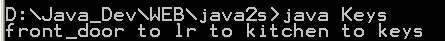8 Compute the area of a triangle using Heron's Formula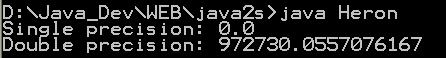9 Compute prime numbers 10 Print a table of fahrenheit and celsius temperatures 1 11 Print a table of fahrenheit and celsius temperatures 2 12 Print a table of Fahrenheit and Celsius temperatures 3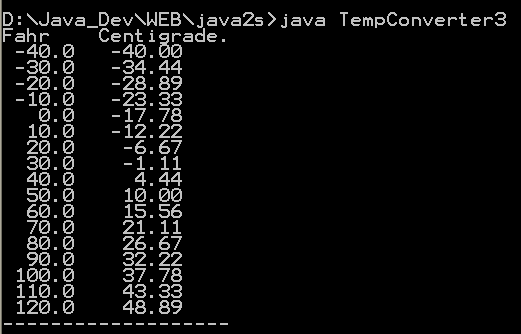13 Soundex - the Soundex Algorithm, as described by Knuth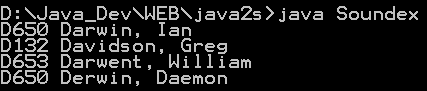14 A programmable Finite State Machine implementation. 15 An extendable Graph datastructure. 16 Utilities for flop (floating-point operation) counting. 17 LU Decomposition 18 Reverse Polish Notation 19 Permutator test 20 implements the LZF lossless data compression algorithm 21 Linear Interpolation 22 Utility class for generating the k-subsets of the numbers 0 to n 23 Version